1. /
2. CBSE
3. /
4. Class 06
5. /
6. Mathematics
7. /
8. NCERT Solutions for Class...

# NCERT Solutions for Class 6 Maths Exercise 5.6

NCERT solutions for Maths Understanding elementary## NCERT Solutions for Class 6 Maths Understanding elementary

###### Question 1.Name the types of following triangles:

(a)Triangle with lengths of sides 7 cm, 8 cm and 9 cm.

(b){tex}\Delta {\text{ABC}}{/tex} with AB = 8.7 cm, AC = 7 cm and BC = 6 cm.

(c){tex}\Delta {/tex}PQR such that PQ = QR = PR = 5 cm.

(d){tex}\Delta {/tex}DEF with {tex}m\angle {/tex}D = {tex}{90^ \circ }{/tex}

(e){tex}\Delta {/tex}XYZ with {tex}m\angle {/tex}Y = {tex}{90^ \circ }{/tex} and XY = YZ

(f){tex}\Delta {/tex}LMN with {tex}m\angle {/tex}L = {tex}{30^ \circ },{/tex}{tex}m\angle {/tex}M = {tex}{70^ \circ }{/tex} and {tex}m\angle {/tex}N = {tex}{80^ \circ }.{/tex}

(a) Scalene triangle

(b) Scalene triangle

(c) Equilateral triangle

(d) Right-angled triangle

(e) Isosceles right-angled triangle

(f) Acute-angled triangle

NCERT Solutions for Class 6 Maths Exercise 5.6

###### Question 2.Match the following:
 Measure of Triangle Types of Triangle (i)3 sides of equal length (a) Scalene (ii) 2 sides of equal length (b) Isosceles right angle (iii) All sides are of different length (c) Obtuse angle (iv) 3 acute angles (d) Right angle (v) 1 right angle (e) Equilateral (vi) 1 obtuse angle (f) Acute angle (vii) 1 right angle with two sidesof equal length (g) Isosceles

Answer: (i) {tex} \to {/tex} (e), (ii) {tex} \to {/tex} (g), (iii) {tex} \to {/tex} (a), (iv) {tex} \to {/tex} (f), (v) {tex} \to {/tex} (d), (vi) {tex} \to {/tex} (c), (vii) {tex} \to {/tex} (b)

###### Question 3.Name each of the following triangles in two different ways: (You may judge the nature of angle by observation)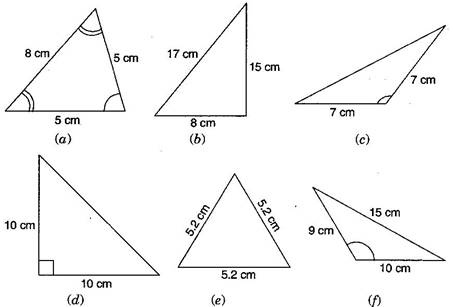(a) Acute angled triangle and Isosceles triangle

(b) Right-angled triangle and Scalene triangle

(c) Obtuse-angled triangle and Isosceles triangle

(d) Right-angled triangle and Isosceles triangle

(e) Equilateral triangle and acute angled triangle

(f) Obtuse-angled triangle and scalene triangle

NCERT Solutions for Class 6 Maths Exercise 5.6

###### Question 4.Try to construct triangles using match sticks. Some are shown here.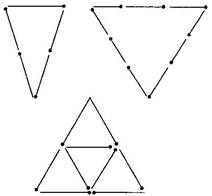Can you make a triangle with:

(a)3 matchsticks?

(b)4 matchsticks?

(c)5 matchsticks?

(d)6 matchsticks?

(Remember you have to use all the available matchsticks in each case)

If you cannot make a triangle, think of reasons for it.

(a) 3 matchsticks

This is an acute angle triangle and it is possible with 3 matchsticks to make a triangle because sum of two sides is greater than third side.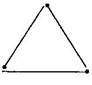(b) 4 matchsticks

This is a square, hence with four matchsticks we cannot make triangle.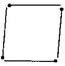(c) 5 matchsticks

This is an acute angle triangle and it is possible to make triangle with five matchsticks, in this case sum of two sides is greater than third side.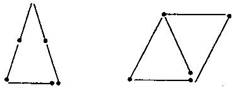(d) 6 matchsticks

This is an acute angle triangle and it is possible to make a triangle with the help of 6 matchsticks because sum of two sides is greater than third side.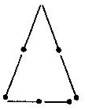## NCERT Solutions for Class 6 Maths Exercise 5.6

NCERT Solutions Class 6 Maths PDF (Download) Free from myCBSEguide app and myCBSEguide website. Ncert solution class 6 Maths includes text book solutions from Class 6 Maths Book . NCERT Solutions for CBSE Class 6 Maths have total 14 chapters. 6 Maths NCERT Solutions in PDF for free Download on our website. Ncert Maths class 6 solutions PDF and Maths ncert class 6 PDF solutions with latest modifications and as per the latest CBSE syllabus are only available in myCBSEguide.

## CBSE app for Students

To download NCERT Solutions for Class 6 Maths, Social Science Computer Science, Home Science, Hindi English, Maths Science do check myCBSEguide app or website. myCBSEguide provides sample papers with solution, test papers for chapter-wise practice, NCERT solutions, NCERT Exemplar solutions, quick revision notes for ready reference, CBSE guess papers and CBSE important question papers. Sample Paper all are made available through the best app for CBSE students and myCBSEguide website.Test Generator

Create question paper PDF and online tests with your own name & logo in minutes.myCBSEguide

Question Bank, Mock Tests, Exam Papers, NCERT Solutions, Sample Papers, Notes

### 2 thoughts on “NCERT Solutions for Class 6 Maths Exercise 5.6”

1. Now we study at home.
Nice.

2. Nice working
Thanks
For
Telling
The